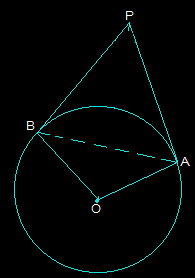Guru

# Prove that the angle between the two tangents drawn from an external point to a circle is supplementary to the angle subtended by the line-segment joining the points of contact at the center. Q.10

• 0

How i prove the angles type question of class 10th ncert book of exercise 10.2 of circles chapter, i don’t know how to solve this problem in easy and simple way give me the best solution for this question Prove that the angle between the two tangents drawn from an external point to a circle is supplementary to the angle subtended by the line-segment joining the points of contact at the center.

Share

1. First, draw a circle with centre O. Choose an external point P and draw two tangents PA and PB at point A and point B respectively. Now, join A and B to make AB in a way that it subtends ∠AOB at the center of the circle. The diagram is as follows:From the above diagram, it is seen that the line segments OA and PA are perpendicular.

So, ∠OAP = 90°

In a similar way, the line segments OB ⊥ PB and so, ∠OBP = 90°

Now, in the quadrilateral OAPB,

∴∠APB+∠OAP +∠PBO +∠BOA = 360° (since the sum of all interior angles will be 360°)

By putting the values we get,

∠APB + 180° + ∠BOA = 360°

So, ∠APB + ∠BOA = 180° (Hence proved).

• 0